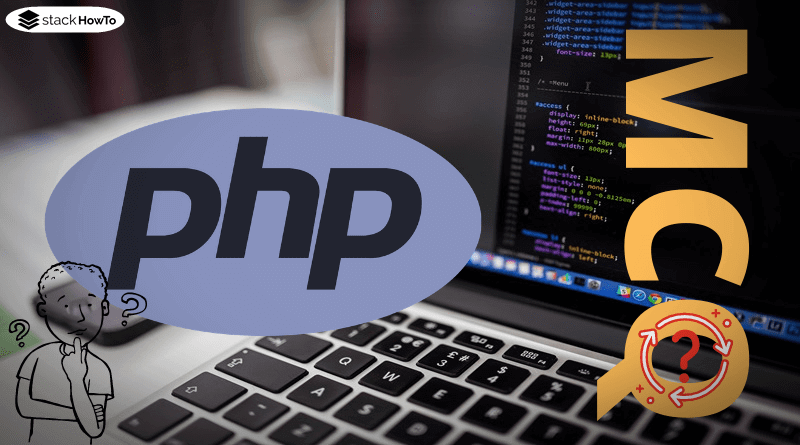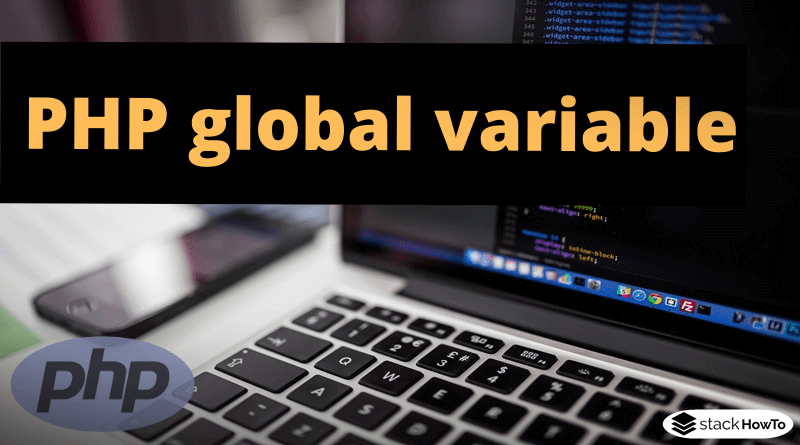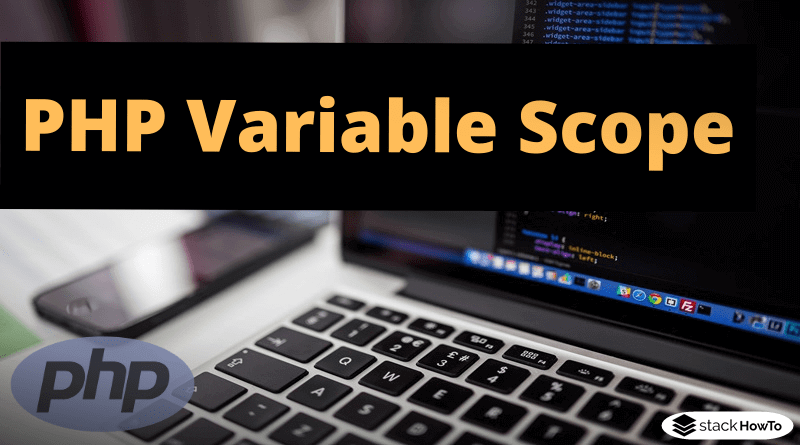php

# How to Define a Variable in PHP

Variables are “containers” that can store specified or determined values, similar to variables in mathematics. These values can be of different types, so-called data types. The most important data types are integer, float, string (words/character, strings or single letters), boolean (truth value, always true or false), and array (a set of values). As opposed to many other programming languages, PHP does not offer the possibility of permanently defining the data type of a variable. Each variable can potentially contain any data type and change it at any time.

Variables always have a name. The characters valid for this name are restricted. In general, only letters from a to z, numbers from 0 to 9, and the underscore may be used. However, numbers can only be used from the second position of the variable name.

var_dump() function can be used for the simple output of variables, which not only shows the content of the variables but also their data types.

###### Example :
```<?php
// Integer number.
\$integer = 50;
var_dump(\$integer);

// Float number.
\$float = 5.25;
var_dump(\$float);

// Strings
\$string = 'Hello';
var_dump(\$string);

// Truth values are represented by "Boolean"
\$boolean = true;
var_dump(\$boolean);

// Arrays can contain one or more values
\$array = array(1, 2, 3, 4, 5, 6);
var_dump(\$array);
?>```

Output:

```int(50)
float(5.25)
string(5) "Hello"
bool(true)
array(6) {
=>
int(1)
=>
int(2)
=>
int(3)
=>
int(4)
=>
int(5)
=>
int(6)
}```PHP MCQ – Multiple Choice Questions and Answers – Basics – Part 1This collection of PHP Multiple Choice Questions and Answers (MCQs): Quizzes & Practice Tests with Answer focuses on “PHP basic”.   1. In PHP, variables…Read More

##### Example : Operations

Below are a few small examples of how to deal with the various data types.

###### Integer and Float :

Mathematical operations on integer variables (can be applied identically to floats):

```<?php
\$a = 10;
\$b = 5;
\$c = \$a + \$b;
var_dump(\$c);
?>```

Output:

`int(15)`

###### Boolean :

An example for the use of truth values or Booleans:

```<?php
\$bool = true;

if (\$bool) {
echo("It's true!");
} else {
echo("It's false!");
}
?>```

Output:

`It's true!`PHP global variable with ExampleVariables usually have a scope, so variables defined in one function are not available for other functions. However, it is also possible to place variables…Read More

###### Strings :

In this example, strings are introduced. The rest of the alphabet is added to the string “abc” in two steps.

```<?php
\$str = "abc";
\$str = \$str . "def";
\$str = \$str . "ghi";
var_dump(\$str);
?>```

Output:

`string(9) "abcdefghi"`

###### Arrays :

In this example, an array is defined, which contains three possible colors. This array is displayed with a short loop (foreach) color by color.

```<?php
\$colors = array('Blue', 'Black', 'Red');

foreach (\$colors as \$color) {
echo("Color : \$color\n");
}
?>```

Output:

```Color : Blue
Color : Black
Color : Red```PHP Variable ScopeWhich areas of application or scopes exist and what effects this has on access to variables? According to the name, the scope of a variable…Read MoreMCQPractice competitive and technical Multiple Choice Questions and Answers (MCQs) with simple and logical explanations to prepare for tests and interviews.Read More